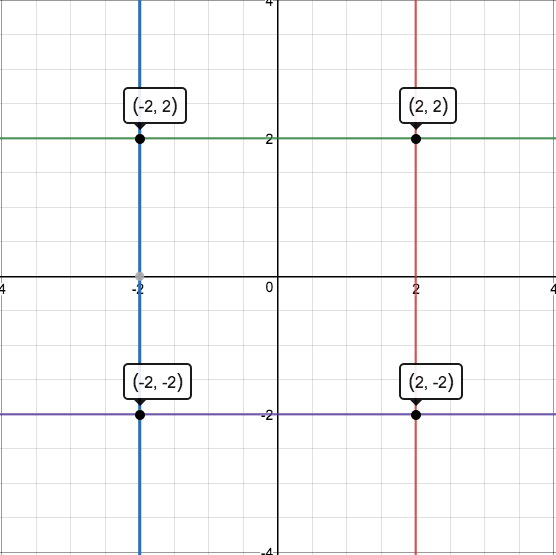Question 21

# In the X-Y plane, the area of the region bounded by the graph of |x+y| + |x-y| = 4 is

Solution

If the moduli are removed, the equations formed are

x+y+x-y = 4 => x=2

x+y-x+y = 4 => y =2

-x-y+x-y = 4 => y=-2

-x-y-x+y = 4 => x=-2

The area enclosed by these equations is a square with vertices at (2,2), (-2,2), (-2,-2), (2,-2) as shown in figure.The required area = 4*4 = 16